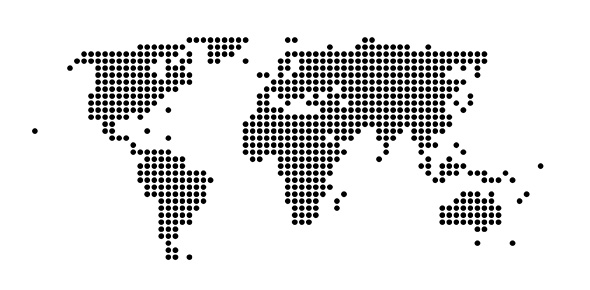# Ied Quiz October 9th - English To Metric, Dial Caliper, Reading Scale

12 QuestionsSettingsThis is a quiz for Intro to engineering design class. Students should be able to convert english to metric, read an english and metric scale, and reading a dial caliper.

• 1.
Why is it important for engineers/designers to be able to convert between the metric system and the english system?  Anwer in complete sentences
• 2.
Convert 76mm to inches:
• A.

7.6 inches

• B.

1930.4 inches

• C.

2.992 inches

• D.

.2992 inches

• 3.
What is the name of this measuring instrument?
• A.

Dial indicator

• B.

Dial caliper

• C.

Rotating ruler

• D.

Engineering scale

• E.

Precision measurer

• 4.
Which of the following is not a measurement that you can perform with the dial caliper?
• A.

Outside diameter/thickness

• B.

Inside diameter

• C.

Depth

• D.

Angle

• E.

Step

• 5.
Convert 3.216 inches to millimeters:
• A.

81.7 mm

• B.

.127 mm

• C.

32.16 mm

• D.

88 mm

• E.

7.9 mm

• 6.
If I have 41 eggs how many dozens do I have?
• A.

3.6 dozen

• B.

4.2 dozen

• C.

3.4 dozen

• D.

492 dozen

• E.

2.5 dozen

• 7.
Is this an englich scale or a metric scale?
• A.

English

• B.

Metric

• 8.
How long is the line?
• A.

2 1/16 inches

• B.

23 mm

• C.

1 5/8 inch

• D.

1 13/16 inches

• E.

1 7/16 inch

• 9.
How long is the line in mm?
• A.

3.4 mm

• B.

34 mm

• C.

23 mm

• D.

3.4 inches

• E.

.34 mm

• 10.
The jaws indicated by the red arrow are used for what type of measurement?
• A.

Outside diameter

• B.

Step measurement

• C.

Angle Measurement

• D.

Inside Diameter

• E.

Whole depth

• 11.
• A.

1.24 inches

• B.

2.09 inches

• C.

5.3 inches

• D.

1346.2 inches

• E.

.479 inches

• 12.
Which precision measuring tool is the most versatile?
• A.

English scale

• B.

Metric scale

• C.

Dial indicator

• D.

Dial caliper

• E.

Engineering scale

More Scale Quizzes
Related Topics